# HSSlive: Plus One & Plus Two Notes & Solutions for Kerala State Board

## BSEB Class 10 Maths Chapter 7 Coordinate Geometry Ex 7.2 Textbook Solutions PDF: Download Bihar Board STD 10th Maths Chapter 7 Coordinate Geometry Ex 7.2 Book AnswersBSEB Class 10 Maths Chapter 7 Coordinate Geometry Ex 7.2 Textbook Solutions PDF: Download Bihar Board STD 10th Maths Chapter 7 Coordinate Geometry Ex 7.2 Book Answers

BSEB Class 10th Maths Chapter 7 Coordinate Geometry Ex 7.2 Textbooks Solutions and answers for students are now available in pdf format. Bihar Board Class 10th Maths Chapter 7 Coordinate Geometry Ex 7.2 Book answers and solutions are one of the most important study materials for any student. The Bihar Board Class 10th Maths Chapter 7 Coordinate Geometry Ex 7.2 books are published by the Bihar Board Publishers. These Bihar Board Class 10th Maths Chapter 7 Coordinate Geometry Ex 7.2 textbooks are prepared by a group of expert faculty members. Students can download these BSEB STD 10th Maths Chapter 7 Coordinate Geometry Ex 7.2 book solutions pdf online from this page.

## Bihar Board Class 10th Maths Chapter 7 Coordinate Geometry Ex 7.2 Books Solutions

 Board BSEB Materials Textbook Solutions/Guide Format DOC/PDF Class 10th Subject Maths Chapter 7 Coordinate Geometry Ex 7.2 Chapters All Provider Hsslive

## How to download Bihar Board Class 10th Maths Chapter 7 Coordinate Geometry Ex 7.2 Textbook Solutions Answers PDF Online?

2. Click on the Bihar Board Class 10th Maths Chapter 7 Coordinate Geometry Ex 7.2 Answers.
3. Look for your Bihar Board STD 10th Maths Chapter 7 Coordinate Geometry Ex 7.2 Textbooks PDF.
4. Now download or read the Bihar Board Class 10th Maths Chapter 7 Coordinate Geometry Ex 7.2 Textbook Solutions for PDF Free.

## BSEB Class 10th Maths Chapter 7 Coordinate Geometry Ex 7.2 Textbooks Solutions with Answer PDF Download

Find below the list of all BSEB Class 10th Maths Chapter 7 Coordinate Geometry Ex 7.2 Textbook Solutions for PDF’s for you to download and prepare for the upcoming exams:

## BSEB Bihar Board Class 10th Maths Solutions Chapter 7 Coordinate Geometry Ex 7.2

Question 1.
Find the coordinates of the point which divides the join of (- 1, 7) and (4, – 3) in the ratio 2:3.
Solution:
Let P(x, y) be the required point.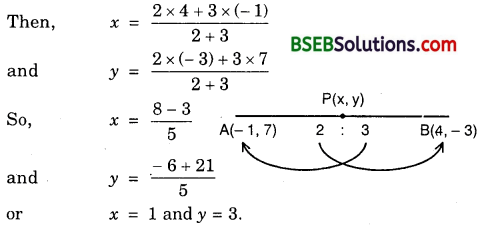So, the coordinates of P are (1, 3).

Question 2.
Find the coordinates of the points of trisection of the lire segment joining (4, – 1) and (- 2, – 3).
Solution:
Let P and Q be the points of trisection of the line segment joining A(4, – 1) and B(- 2, – 3). Then, AP = PQ = QB = k (say).
∴ PB = PQ + QB = 2k
and AQ = AP + PQ = 2k
So, AP : PB = k : 2k = 1 : 2 and AQ : QB = 2k : k = 2 : 1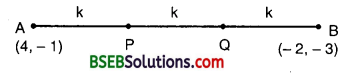So, P divides AB internally in the ratio 1 : 2, while Q divides AB internally in the ratio 2 : 1. Thus, the coordinates of P are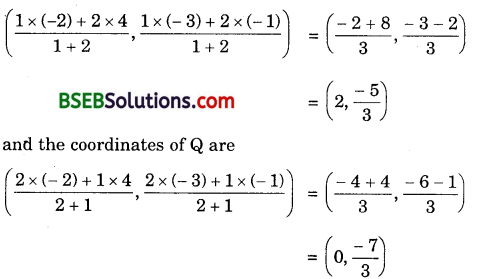Hence, the two points of trisection are
(2, −53) and (0, −73).

Question 3.
To conduct Sports Day activities in your rectangular shaped school ground ABCD, lines have been drawn with chalk powder at a distance of 1 m each. 100 flower pots have been placed at a distance of 1 m from each other along AD, as shown in figure.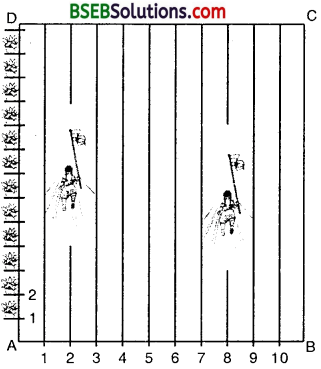Niharika runs 14 th the distance AD on the 2nd line and posts a green flag. Preet runs 15 th the distance AD on the eighth line and posts a red flag. What is the distance between both the flags ? If Rashmi has to post a blue flag exactly halfway between the line (segment) joining the two flags, where should she post her flag ?
Solution:
Clearly from the figure, the position of green flag posted by Niharika is given by P(2, 14 x 100) i.e., P(2, 25) and that of red, flag posted by Preet is given by Q(8, 15 x 100), i.e., Q(8, 20)
Now, PQ = (8−2)2+(20−25)2‾‾‾‾‾‾‾‾‾‾‾‾‾‾‾‾‾‾‾‾√ = (6)2+(−5)2‾‾‾‾‾‾‾‾‾‾‾‾√
= 36+25‾‾‾‾‾‾‾‾√
= 61‾‾‾√
∴ The distance between the flags = 61‾‾‾√ metres
Let M be the position of the blue flag posted by Rashmi in the halfway of line segment PQ.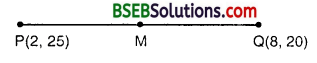∴ M is the given by (2+82,25+202)
or (102,452), i.e., (5, 22.5)
Thus, the blue flag should be on the fifth line at a distance of 22.5 m above it.

Question 4.
Find the ratio in which the line segment joining the points (- 3, 10) and (6, – 8) is divided by (- 1, 6).
Solution:
Let the point P(- 1, 6) divide the line joining A(- 3, 10) and B(6, – 8) in- the ratio k : 1. Then, the coordinates of P are (6𝑘−3𝑘+1,−8𝑘+10𝑘+1)
But, the coordinates of P are given as (- 1, 6).Hence, the point P divides AB in the ratio 2 : 7.

Question 5.
Find the ratio in which the line segment joining A(1, – 5) and B(- 4, 5) is divided by the x-axis. Also, find the coordinates of the point of division.
Solution:
Let the required ratio be k : 1. Then, the coordinates of the point P of division are (−4𝑘+1𝑘+1,5𝑘−5𝑘+1)But it is a point on x-axis on which y-coordinate of every point is 0.
∴ 5𝑘−5𝑘+1 = 0
or 5k – 5 = 0
5k = 5
k = 1
Thus, the required ratio is 1 : 1 and the point of division P is given by
(−4×1+11+1,0), i.e., (−4+12,0) i.e., (−32,0)

Question 6.
If (1, 2), (4, y), (x, 6) and (3, 5) are the vertices of a parallelogram taken in order, find x and y.
Solution:
Let A(1, 2), B(4, y), C(x, 6) and D(3, 5) be the vertices of a parallelogram ABCD.
Since the diagonals of a parallelogram bisect each other, therefore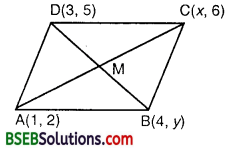𝑥+12 = 3+42
or x + 1 = 7 or x = 6
and 5+𝑦2 = 6+22
or 5 + y = 8
or y = 3
Hence, x = 6 and y = 3.

Question 7.
Find the coordinates of a point A, where AB is the diameter of a circle whose centre is (2, – 3) and B is (1, 4).
Solution:
Let AB be a diameter of the circle having its centre at C(2, – 3) such that the coordinates of end B are (1, 4).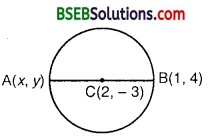Let the coordinates of A be (x, y).
Since C is the mid-point of AB, therefore
the coordinates of C are (𝑥+12,𝑦+42)
But, the coordinates of C are given as (2, – 3).
∴ 𝑥+12 = 2 or x + 1 = 4 or x = 3
and 𝑦+42 = – 3 or y + 4 = – 6 or y = – 10
Hence, the coordinates of A are (3, – 10).

Question 8.
If A and B are (- 2, – 2) and (2, – 4) respectively, find the coordinates of P such that AP = lies on the line segment AB.
Solution:
We have : AP = 37ABLet P(x, y) be the point which divides the join of A(- 2, – 2) and B(2, – 4) in the ratio 3:4.Hence, the coordinates of the point P are $$. Question 9. Find the coordinates of the points which divide the line segment joining A(- 2, 2) and B(2, 8) into four equal parts. Solution: Let P1 P2 and P3 be the points that divide the line segment joining A(- 2, 2) and B(2, 8) into four equal parts.Since P1 divides the line segment into two equal parts, therefore coordinates of P2 (i.e., mid-point) are [latex]\left(\frac{-2+2}{2}, \frac{2+8}{2}\right)$$, i.e., (0, 5).
Now, P1 divides the line segment AP2 into two equal parts.
Coordinates of P1 (i.e., mid-point) are (−2+02,2+52), i,e., (−1,72),
Again, P3 is the mid-point of line segment P2B.
∴ Coordinates or P3 are (0+22,5+82), i.e., (1, 132).

Question 10.
Find the area of a rhombus if its vertices are (3, 0), (4, 5), (- 1, 4) and (- 2, – 1) taken in order.
Solution:
Let A(3, 0), B(4, 5), C(- 1, 4) and D(- 2, – 1) be the vertices of the rhombus ABCD.## Bihar Board Class 10th Maths Chapter 7 Coordinate Geometry Ex 7.2 Textbooks for Exam Preparations

Bihar Board Class 10th Maths Chapter 7 Coordinate Geometry Ex 7.2 Textbook Solutions can be of great help in your Bihar Board Class 10th Maths Chapter 7 Coordinate Geometry Ex 7.2 exam preparation. The BSEB STD 10th Maths Chapter 7 Coordinate Geometry Ex 7.2 Textbooks study material, used with the English medium textbooks, can help you complete the entire Class 10th Maths Chapter 7 Coordinate Geometry Ex 7.2 Books State Board syllabus with maximum efficiency.

## FAQs Regarding Bihar Board Class 10th Maths Chapter 7 Coordinate Geometry Ex 7.2 Textbook Solutions

#### Can we get a Bihar Board Book PDF for all Classes?

Yes you can get Bihar Board Text Book PDF for all classes using the links provided in the above article.

## Important Terms

Bihar Board Class 10th Maths Chapter 7 Coordinate Geometry Ex 7.2, BSEB Class 10th Maths Chapter 7 Coordinate Geometry Ex 7.2 Textbooks, Bihar Board Class 10th Maths Chapter 7 Coordinate Geometry Ex 7.2, Bihar Board Class 10th Maths Chapter 7 Coordinate Geometry Ex 7.2 Textbook solutions, BSEB Class 10th Maths Chapter 7 Coordinate Geometry Ex 7.2 Textbooks Solutions, Bihar Board STD 10th Maths Chapter 7 Coordinate Geometry Ex 7.2, BSEB STD 10th Maths Chapter 7 Coordinate Geometry Ex 7.2 Textbooks, Bihar Board STD 10th Maths Chapter 7 Coordinate Geometry Ex 7.2, Bihar Board STD 10th Maths Chapter 7 Coordinate Geometry Ex 7.2 Textbook solutions, BSEB STD 10th Maths Chapter 7 Coordinate Geometry Ex 7.2 Textbooks Solutions,
Share: